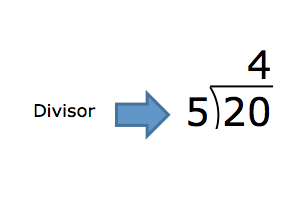Quotient

My Definition

Key Characteristics

The following are true of a quotient:

• It is the result of a division problem.

• It may be a whole number, a whole number and a remainder, or a rational number.

• It may identify the number of groups in a given amount when the number in each group is known when using the partitive method of division.

• It may identify the number in each group when the number of groups within a given amount is known when using the quotative method of division.

Example

Use the link below to further study examples of a quotient.

Quotient Activity

Non-exampleTEKS: 3(4)(J); 4(4)(E); 5(3)(C); 5(3)(F); 5(3)(G); 6(4)(D); A2(7)(C); A2(7)F); AR(4)(C)